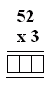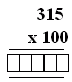Name: ___________________Date:___________________

 Email us to get an instant 20% discount on highly effective K-12 Math & English kwizNET Programs!

### MEAP Preparation - Grade 5 Mathematics1.45 Multiplication Pictures

 Method: When multiplying two numbers, consider the first number. The first number is multiplied by each of the digits of the second number. Add the products. Example: 12 x 13 = ?Multiply 12 and 3(always start with ones). Multiply 12 and 1. (this is shifted one place to left) Add the products. Answer: 156 Directions: Multiply the following. Also write at least ten examples of your own.
 Q 1: Find the product of the multiplication.156155160 Q 2: Find the product of the multiplication.315315031500 Q 3: Find the product of the multiplication.423042004202 Q 4: Find the product of the multiplication.225250252 Question 5: This question is available to subscribers only! Question 6: This question is available to subscribers only!